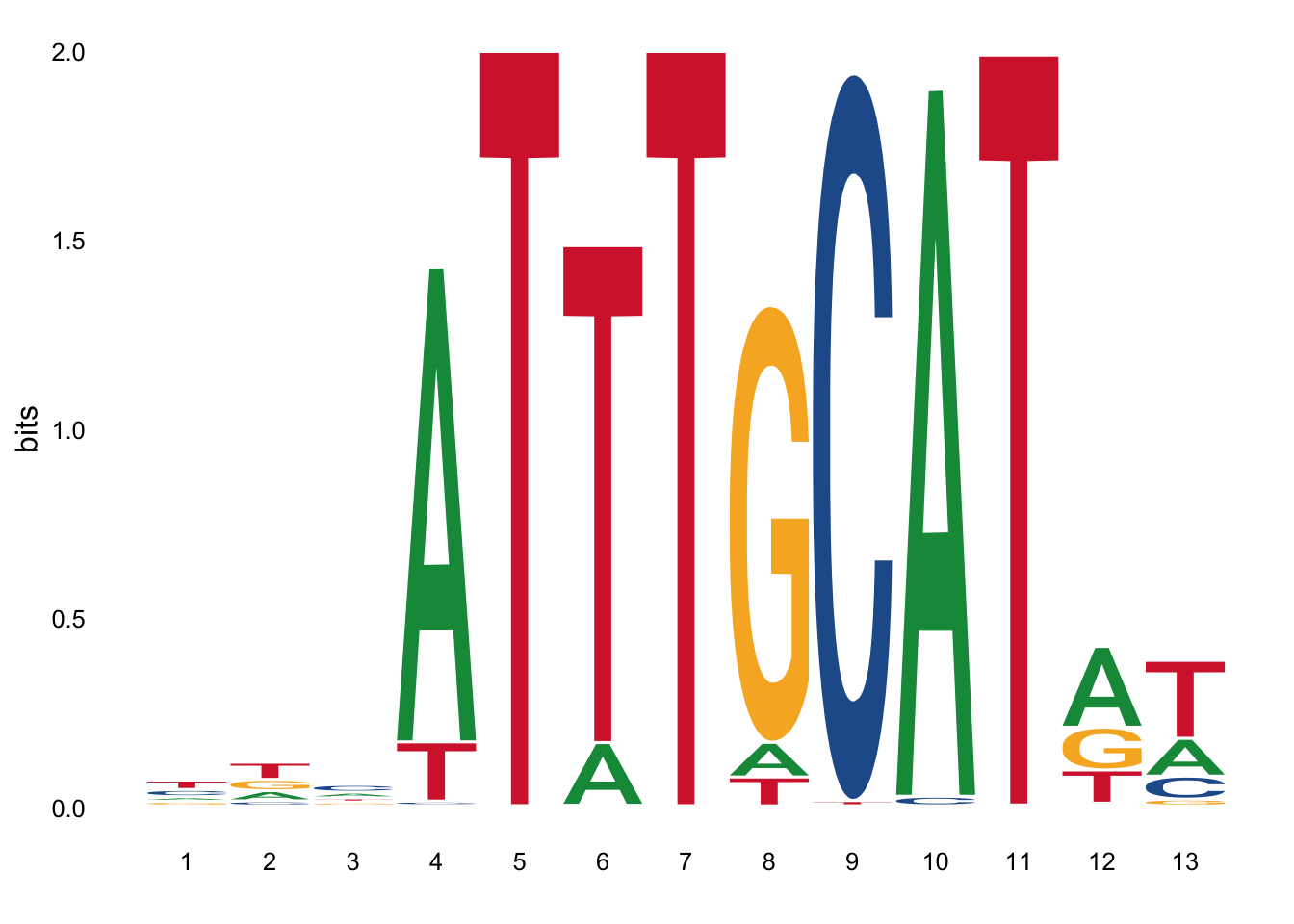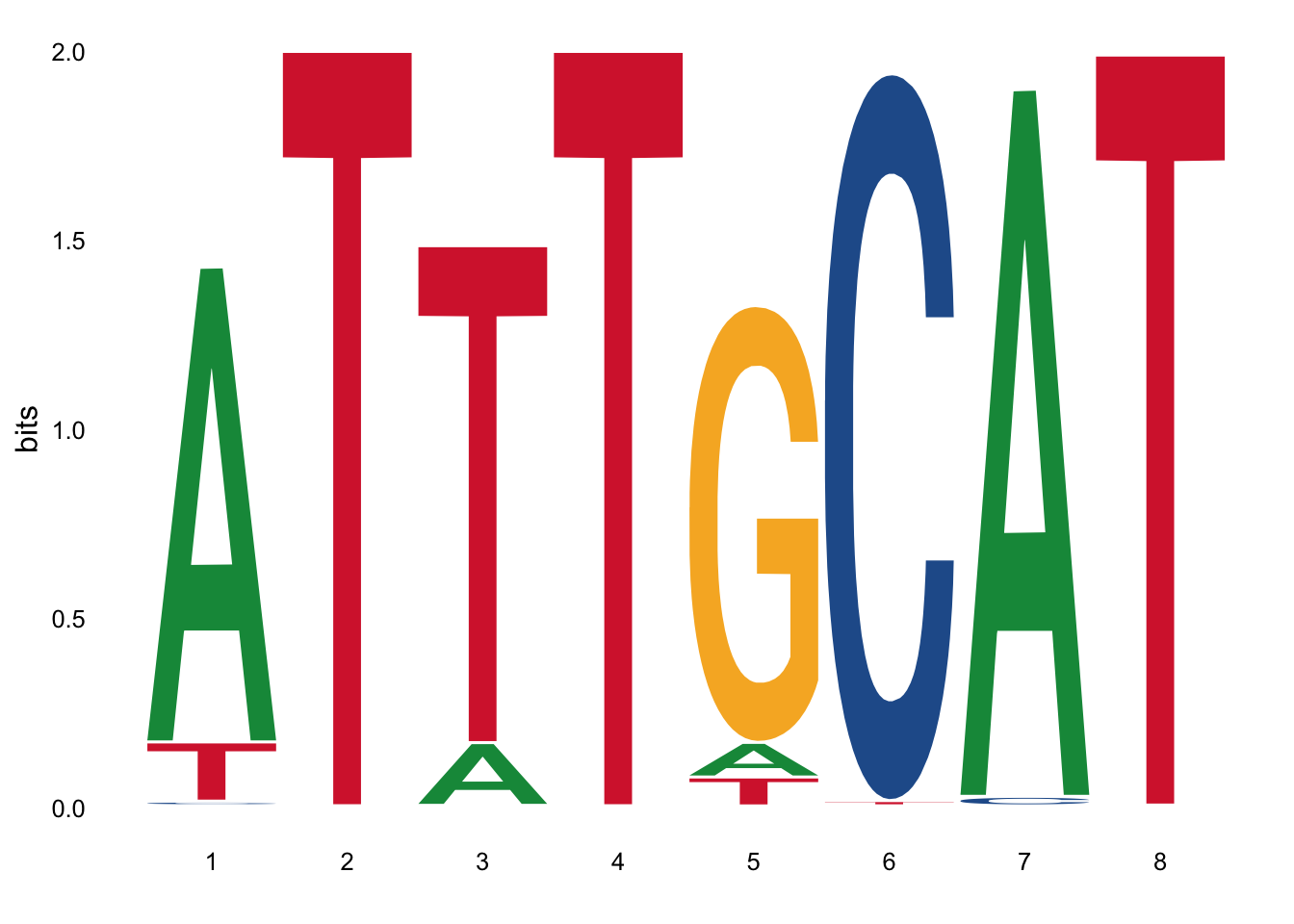# Introduction

The following script represents the transcription factor motif-based analysis performed in our recent paper “An OCT2 / OCA-B / MEF2B Ternary Complex Controls the Activity and Architecture of an Essential Locus Control Region for Normal and Malignant Germinal Center B-cells”. This script runs through the following steps for the two TFs we are focusing on, OCT2 (POU2F2) and MEF2B :

• trim motifs to remove low information content
• convert the basic Position Count Matrix (PCM) to Position Weight Matrix (PWM)
• search genomic region of interest for matches to PWM motif
• calculate p values for each match

The data generated here (i.e. TF motif matches to the BCL6 locus) are used in the analysis of TF motif density in essential vs non-essential enhancers described in a separate script.
The script is extensively annotated and can thus be used as a hands-on tutorial for TF motif analysis. Please feel free to email me with any questions, comments or suggestions.
info at jchellmuth.com

``````library(universalmotif)
library(JASPAR2018)
library(BSgenome.Hsapiens.UCSC.hg38)
library(dplyr)
library(ggplot2)``````

### Get the genomic sequence for the region of interest

Note on nomenclature: this is the ‘search subject’, i.e. the region to be searched for a given motif.
The region of interest here is the BCL6 locus, losely defined as:
chr3:187,700,000-188,300,000

``````hg38 <- BSgenome.Hsapiens.UCSC.hg38
chr3 <- getSeq(hg38,"chr3")
roi <- subseq(chr3,start=187700000,end=188300000)``````

# OCT2 motif analysis

http://jaspar.genereg.net/matrix/MA0507.1/

``````# import motif from file:
pfm <- TFBSTools::getMatrixByID(x = JASPAR2018,ID = "MA0507.1")``````
``## ``
``````# check key tags
pfm@tags[c("symbol","description","collection","type","source","species")]``````
``````## \$symbol
##  "POU2F2"
##
## \$description
##  "POU class 2 homeobox 2"
##
## \$collection
##  "CORE"
##
## \$type
##  "ChIP-seq"
##
## \$source
##  "ENCODE"
##
## \$species
##           9606
## "Homo sapiens"``````
``````# convert to universalmotif class for easier handling
pfm <- convert_motifs(pfm,class = "universalmotif-universalmotif")``````

### Trim motif

Motifs frequently contain positions with low information content (see non-trimmed motif below). Here, I am using universalmotif’s `trim_motif` to remove edges of motifs with an information content <0.5

``````# visualize full motif
view_motifs(pfm)````````````# trim
pfm.trim <- trim_motifs(pfm,min.ic = 0.5)``````
``````# visualize trimmed motif:
view_motifs(pfm.trim)``````### Motif representations

There are 3 commonly used TF motif representations:

• Position Frequncy Matrix (PFM) aka Postion Count Matrix (PCM)
• Information Content Matrix (ICM)
• Postion Weight Matrix (PWM) aka Logodds Scoring Matrix

Each of these is informative in a different way and is therefore displayed below.

#### Position Frequency Matrix (PFM) aka Postion Count Matrix (PCM)

This is the most basic representation of a motif and is how the motif is provided by Jaspar.

``knitr::kable(pfm.trim@motif,right = F)``
A T T T G C A T
A 2016 0 256 0 158 0 2257 0
C 19 0 2 0 0 2271 30 2
G 1 0 0 0 1997 0 0 0
T 251 2287 2029 2287 132 16 0 2285

#### Information Content Matrix (ICM)

ICMs are usually used to represent the motif as a probability sequence logo (as above). The height of the letters represent their probabilities at each position. The ICM has a column sum between 0 (no base preference) and 2 (only 1 base used).

``````icm <- convert_type(pfm.trim,type = "ICM")
knitr::kable(icm@motif)``````
A T T T G C A T
A 1.2560832 0.000218 0.1659172 0.000218 0.0915148 0.0002115 1.8698794 0.0002170
C 0.0119924 0.000218 0.0014568 0.000218 0.0001446 1.9217363 0.0250587 0.0019526
G 0.0007787 0.000218 0.0001619 0.000218 1.1549947 0.0002115 0.0002071 0.0002170
T 0.1565237 1.994559 1.3139025 1.994559 0.0764792 0.0137494 0.0002071 1.9831807

#### Postion Weight Matrix (PWM) aka Logodds Scoring Matrix

PWMs are generally used to evaluate how well a sequence matches a motif. Thus, use PWM for `scan_sequences` below.
Following Nishida et al. Nucleic Aciids Research 2009, a pseudocount of 0.8 is added for conversion to PWM.

``````pwm <- convert_type(pfm.trim,type = "PWM",pseudocount = 0.8)
knitr::kable(pwm@motif)``````
A T T T G C A T
A 1.817678 -11.481673 -1.158619 -11.481673 -1.854139 -11.481673 1.980573 -11.481673
C -4.896711 -11.481673 -8.022242 -11.481673 -11.481673 1.989494 -4.243269 -8.022242
G -8.896711 -11.481673 -11.481673 -11.481673 1.804018 -11.481673 -11.481673 -11.481673
T -1.187053 1.999622 1.826950 1.999622 -2.113167 -5.141823 -11.481673 1.998360

### Search for motif in region of interest

From the universalmotif vignette: Given a motif of length n, scan_sequences() considers every possible n-length subset in a sequence and scores it using the PWM format. If the match surpasses the minimum threshold, it is reported.
For now, I am using a low threshold. Which thershold / match to consider significant is a complex question and will be dealt with in downstream analysis.

``````# scan sequence for matches:
res <- scan_sequences(pwm,DNAStringSet(roi),threshold = 0.75,threshold.type = "logodds",RC = T,verbose = 0)``````

Correct match coordinates by adding the genomic coordinate of the start pos of the region of interest. and add chromosome name.

``````res\$start <- res\$start+187700000-1
res\$stop <- res\$stop+187700000-1
res\$sequence <- "chr3"``````

### Calculate p-values

P-value calculation as per universalMotif Vignette:
https://bioconductor.org/packages/release/bioc/vignettes/universalmotif/inst/doc/MotifComparisonAndPvalues.pdf
Note: smaller k leads to faster but worse approximations, and larger k leads to slower but better approximations. If k is equal to the width of the motif, then the calculation is exact.

``````# get background nucleotide frequency:
bkg <- Biostrings::oligonucleotideFrequency(roi,width = 1,as.prob = T)
# calculate p values:
res\$pvalue <- motif_pvalue(pwm, res\$score, bkg.probs = bkg,k = 8)``````

### Modify results object

For minus strand hits produced by `scan_sequences`, the ‘start’ position of after the ’end position. To make these genomic positions work in bed files and UCSC, I will flip start and stop positions for minus strand hits.

``````minus <- res\$strand=="-"
res[minus, c("start", "stop")] <- res[minus, c("stop", "start")]``````

Similarly, add the reverse complement of hits on the minus strand.

``````res\$match.stranded <- res\$match
res\$match.stranded[minus] <- reverseComplement(DNAStringSet(res\$match.stranded[minus]))``````

Save results for later analysis

``````# save results:
save(pwm,file = "OCT2_MA0507.1_PWM.Rda")
save(res,file = "BCL6locus-matches_OCT2_MA0507.1.Rda")``````

### Cutoff evaluation

The question of which match is to be considered significant or biologically relevant is difficult to answer and will depend on things like the type of analysis, the TF at hand or chromatin context.
The following chunk is meant to give a rough overview of how relative match scores (`score.pct` in the scan_sequences results) relate to PWM scores and p-values (an vice versa).

``````df <- data.frame(rel.score=c(0.75,0.8,0.85,0.9,0.95,0.99,1))
df\$pwm.score <- motif_score(pwm,df\$rel.score)
df\$pvalue <- motif_pvalue(pwm,score = df\$pwm.score)
knitr::kable(df)``````
rel.score pwm.score pvalue
0.75 -10.75675 0.0082550
0.80 -5.52300 0.0044250
0.85 -0.28925 0.0010376
0.90 4.94450 0.0003815
0.95 10.17825 0.0000763
0.99 14.36525 0.0000153
1.00 15.41200 0.0000000

The following chunk gives you example matches at different threshold.

``````res\$cut <- cut(res\$score.pct,breaks = c(75,80,85,90,95,99,100))
res %>% group_by(cut) %>% top_n(-1,score.pct) %>% filter(!duplicated(score.pct)) %>% arrange(score.pct) %>% ungroup() %>% select(-cut) %>% knitr::kable()``````
motif sequence start stop score max.score score.pct match strand pvalue match.stranded
POU2F2 chr3 187700654 187700661 -9.955 15.41557 75.21742 TTAGGCCT + 0.0135559 TTAGGCCT
POU2F2 chr3 187749361 187749368 -5.058 15.41557 80.00093 GTCTGCAT + 0.0071330 GTCTGCAT
POU2F2 chr3 187717154 187717161 0.842 15.41557 85.76419 GTTTTCAT + 0.0018727 GTTTTCAT
POU2F2 chr3 187711693 187711700 5.275 15.41557 90.09445 ATTTTCCT + 0.0006622 ATTTTCCT
POU2F2 chr3 187740876 187740883 11.496 15.41557 96.17127 ATTTTCAT + 0.0001145 ATTTTCAT
POU2F2 chr3 187707731 187707738 15.411 15.41557 99.99553 ATTTGCAT + 0.0000262 ATTTGCAT

# MEF2B motif analysis

``````# import motif from file:
pfm <- TFBSTools::getMatrixByID(x = JASPAR2018,ID = "MA0660.1")
# check key tags
pfm@tags[c("remap_tf_name","collection","type","source","species")]``````
``````## \$remap_tf_name
##  "MEF2B"
##
## \$collection
##  "CORE"
##
## \$type
##  "HT-SELEX"
##
## \$source
##  "23332764"
##
## \$species
##           9606
## "Homo sapiens"``````
``````# convert to universalmotif class for easier handling
pfm <- convert_motifs(pfm,class = "universalmotif-universalmotif")``````

### Trim motif

``````# visualize full motif
view_motifs(pfm)``````